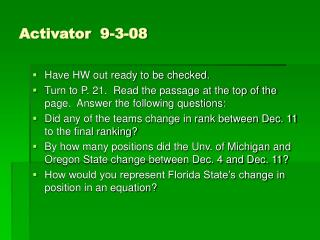DownloadDownload PresentationActivator 9-3-08

# Activator 9-3-08

Télécharger la présentation## Activator 9-3-08

- - - - - - - - - - - - - - - - - - - - - - - - - - - E N D - - - - - - - - - - - - - - - - - - - - - - - - - - -
##### Presentation Transcript

1. Activator 9-3-08 • Have HW out ready to be checked. • Turn to P. 21. Read the passage at the top of the page. Answer the following questions: • Did any of the teams change in rank between Dec. 11 to the final ranking? • By how many positions did the Unv. of Michigan and Oregon State change between Dec. 4 and Dec. 11? • How would you represent Florida State’s change in position in an equation?

2. How are the properties of identity and equality used? • Additive Identity - the sum of any number and 0 is equal to the number. • a + 0 = a 0 + 5 = 5 • Multiplicative Identity - the product of any number and 1 is equal to the number. • a * 1 = a 12 * 1 = 12 • Multiplicative Property of Zero - the product of any number and zero is zero. • n * 0 = 0 15 * 0 = 0

3. Properties continued - • Multiplicative Inverses or reciprocals – two numbers whose product is equal to 1. • Write the fraction two thirds, and then write its reciprocal. • Name the property used in each equation. Then find the value of n. • A. n * 12 = 0 • B. n * 1/5 = 1 • C. 0 + 8 = 8

4. Properties of equality that apply to addition and multiplication Reflexive – any quantity is equal to itself. 7 = 7 2 + 3 = 3 + 2 Symmetric – If one quantity equals a second quantity, then the second quantity equals the first. If 9 = 6 + 3 then 6 + 3 = 9 Transitive – if one quantity equals a second quantity and the second quantity equals a third quantity, then the first quantity equals the third quantity. If a = b and b = c, then a = c If 5 + 7 = 8 + 4 and 8 + 4 = 12 then 5 + 7 equals 12

5. Substitution – A quantity can be substituted for its equal in any expression. • If a = b then it may be replaced by b in any expression. • If n = 15, then 3n = 3 * 15. • The properties of identity and equality can be used to justify each step when evaluating an expression. • Evaluate 2(3 * 2 – 5) + 3 * 1 . Name each property • 3 used in each step.

6. Your Turn!  Evaluate 1 (12 – 8) + 3(15 / 5 – 2). • 4 • Name the property used in each step.

7. Summarizer • Get out a clean sheet of paper and turn it landscape style. • You are going to construct a Tree Map of the properties. • Title – Properties • Branches – Reflexive, Symmetric, Transitive, Substitution. • List five examples under each branch. • When complete, turn in on the front table and begin working on homework.